# RC Circuit Analog Interview Questions | 2R-2C Combination Step Voltage Input

In my previous post RC Circuit Analysis , I have described the fundamentals of simple Analog RC circuit which is the backbone of all other RC circuit combination analysis. I highly recommend to go through the previous post RC Circuit Analysis to get familiarize with fundamentals of RC analysis.

In this post we are going discuss the behavior of 2R-2C RC circuit excited with step voltage input. In our analysis all the components are considered ideal.

The 2R-2C combination RC circuit is given below.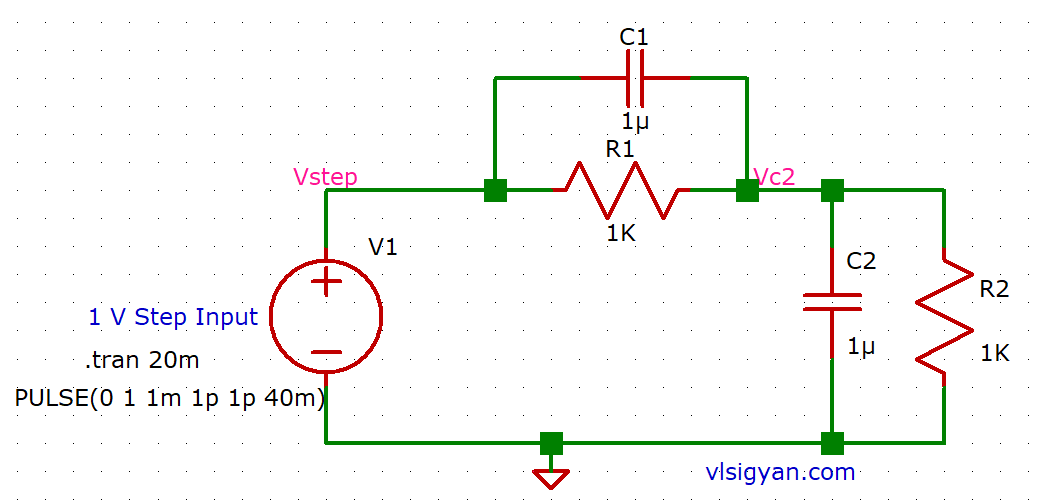From our earlier analysis in RC Circuit Analysis , we are aware that we need to find out the voltage across capacitor and resistor and current through them at time t=0+ and t=infinity.

#### Analysis at time t=0+

Now, for the above circuit, before application of voltage inputs capacitors are fully discharged (voltage across them= 0 v). After application of step voltage, from  the fundamental property of capacitors, the capacitor will prevent the sudden change of the voltage across it and will try to remain at voltage=0. But, for the above circuit, considering all the elements are ideal, a unique incident takes place.

From our previous analysis, atthe circuit will look like below.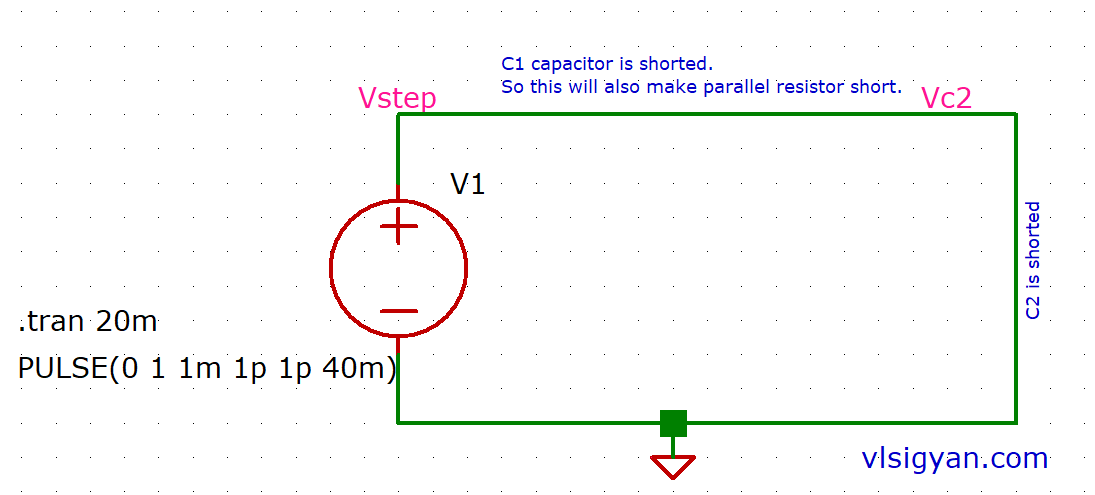The above circuit at t=0+ violates Kirchhoff’s voltage law (KVL).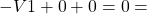>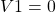, but we know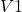is not zero at.

To satisfy KVL, the voltage across capacitor (capacitor charge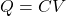)  gets distributed atand capacitor voltages changes abruptly at( all ideal capacitors). Now, to change voltage across capacitor we need infinite amount of current or in a word we need impulse current.  From the fundamental i-v equation of capacitor, capacitor integrates  current to get the voltage. So when impulse current gets integrated we get step voltage which changes the voltage across capacitor abruptly at. This gives us the voltage across capacitor at. Below is the circuit, at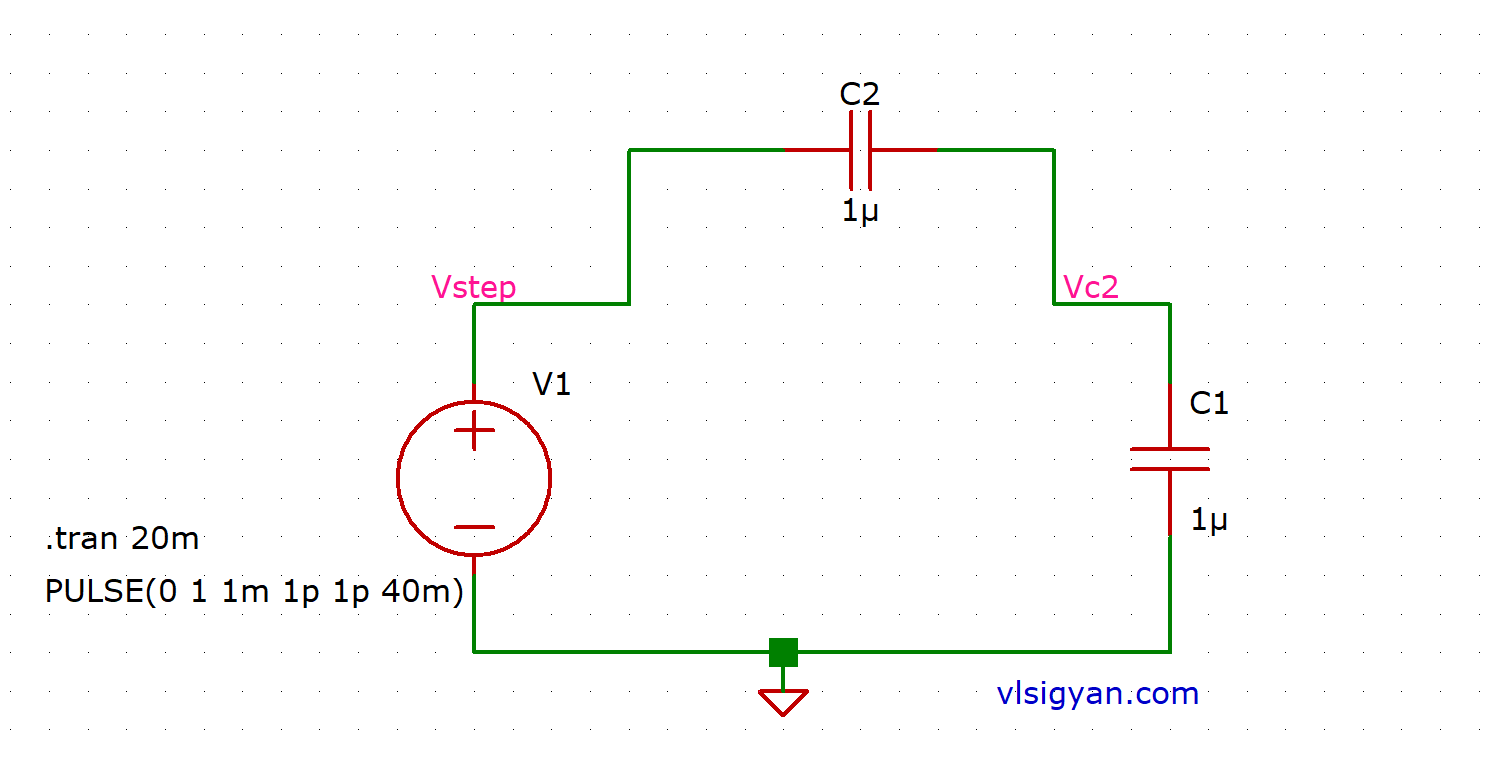From the voltage divider formula of capacitors in series, we can write

Voltage across capacitor C1,Voltage across capacitor C2,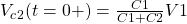#### Analysis at time t=infinity

From our previous post with 1R-1C circuit, we know that when capacitor is fully charged it acts like an open circuit and no current flows through it.  As in this circuit two capacitors are there we need to find out the what would be the voltage at at t=infinity. We need  to keep in mind that KCL and KVL should satisfy at each point of our analysis of RC circuits.

Keeping this in mind, at t=infinity, let’s say capacitor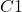and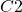charges fully, behaves as an open circuit and current flowing through them is zero. So, we now basically have a circuit with two series resistances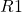and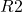. As this is a series circuit, the same current will be flowing through both the resistors.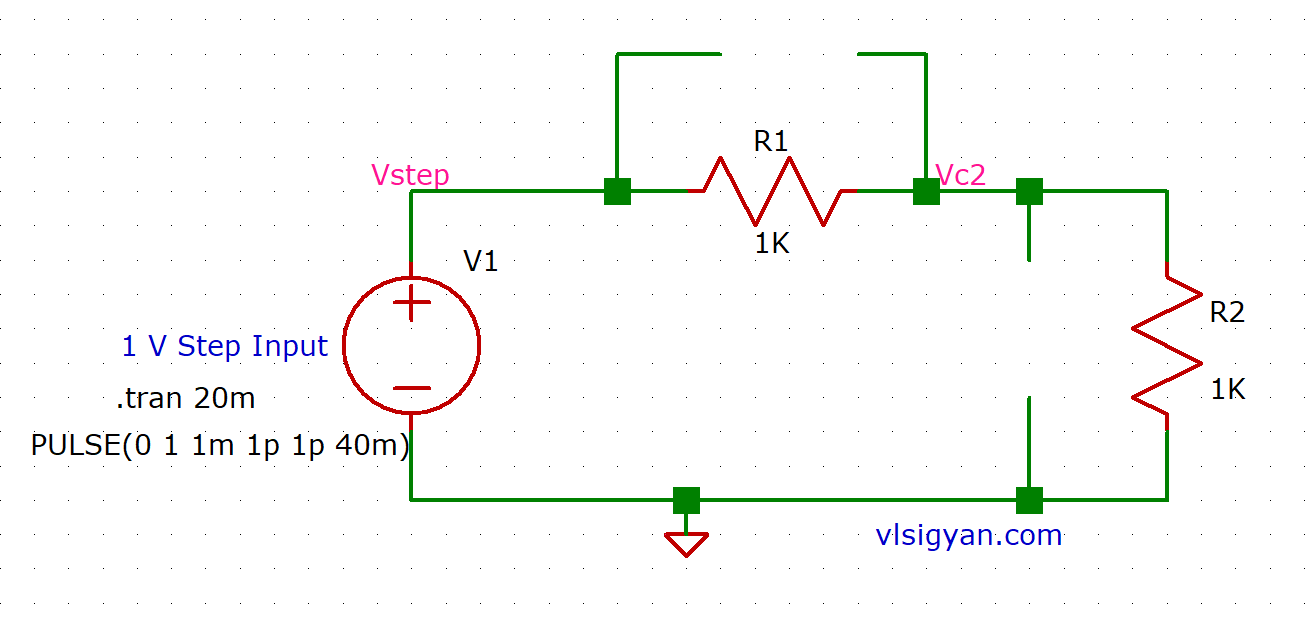This gives us the voltage across R1 at t=infinity, from voltage divider rule,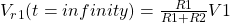Voltage across R2 at t=infinity, using KVL,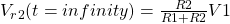If R1=R2, then the voltage across the resistors become half of input voltage (at steady state). i.e,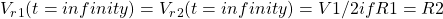The time constant of circuit can be calculated by shorting the voltage source and calculating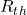and.

Here,,  time constant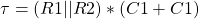#### Important Observation for this type of 2R-2C Circuit:

If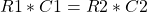,then the output (voltage across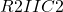)will be attenuated equally at t=0+ and t=infinity. This because, the o/p voltage depends on the ratio of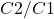at t=0+ and on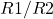at t=infinity, When, the ratio multiplied to input to get o/p voltage becomes equal.

Below shows the simulation result in LTspice.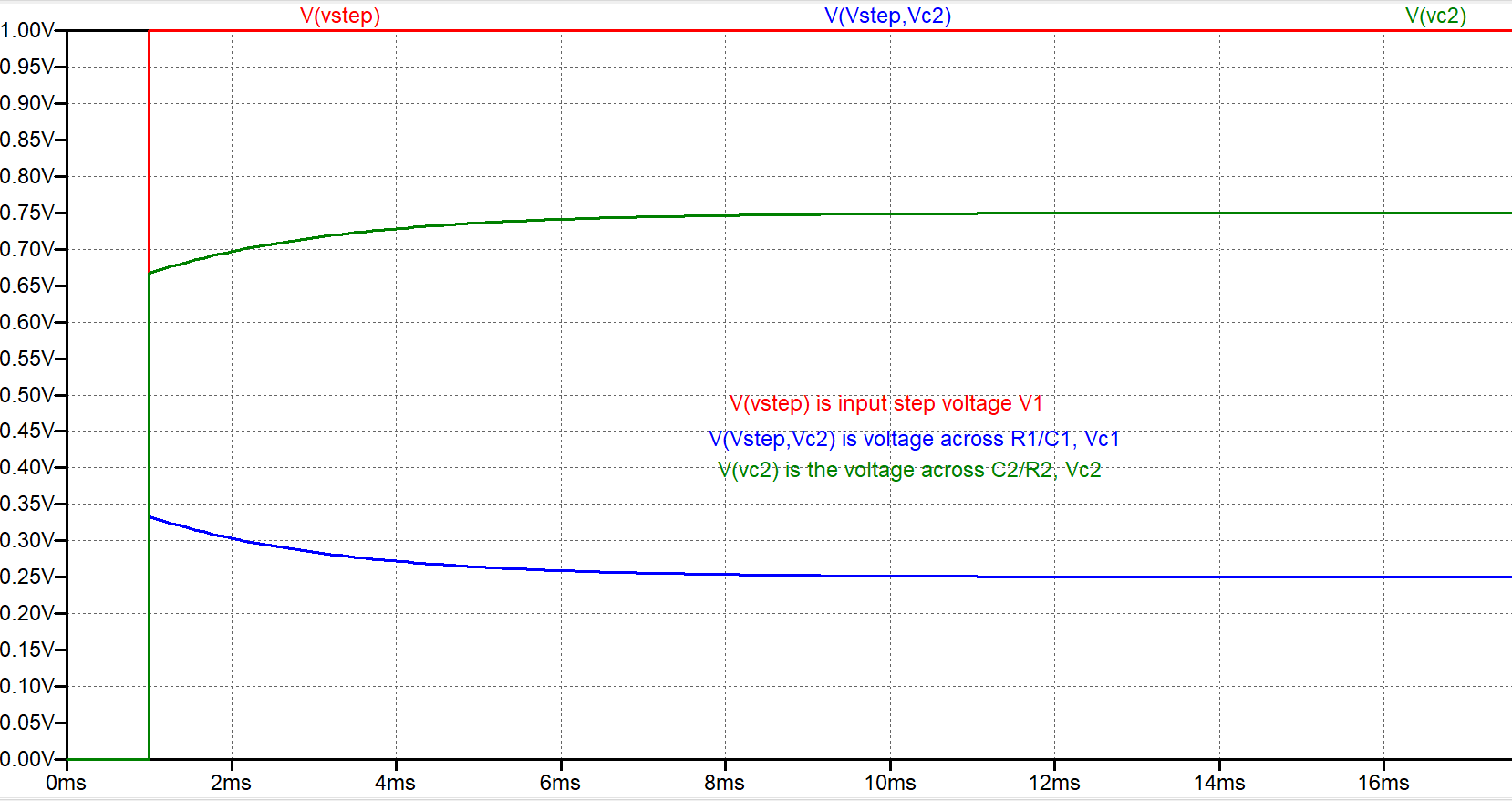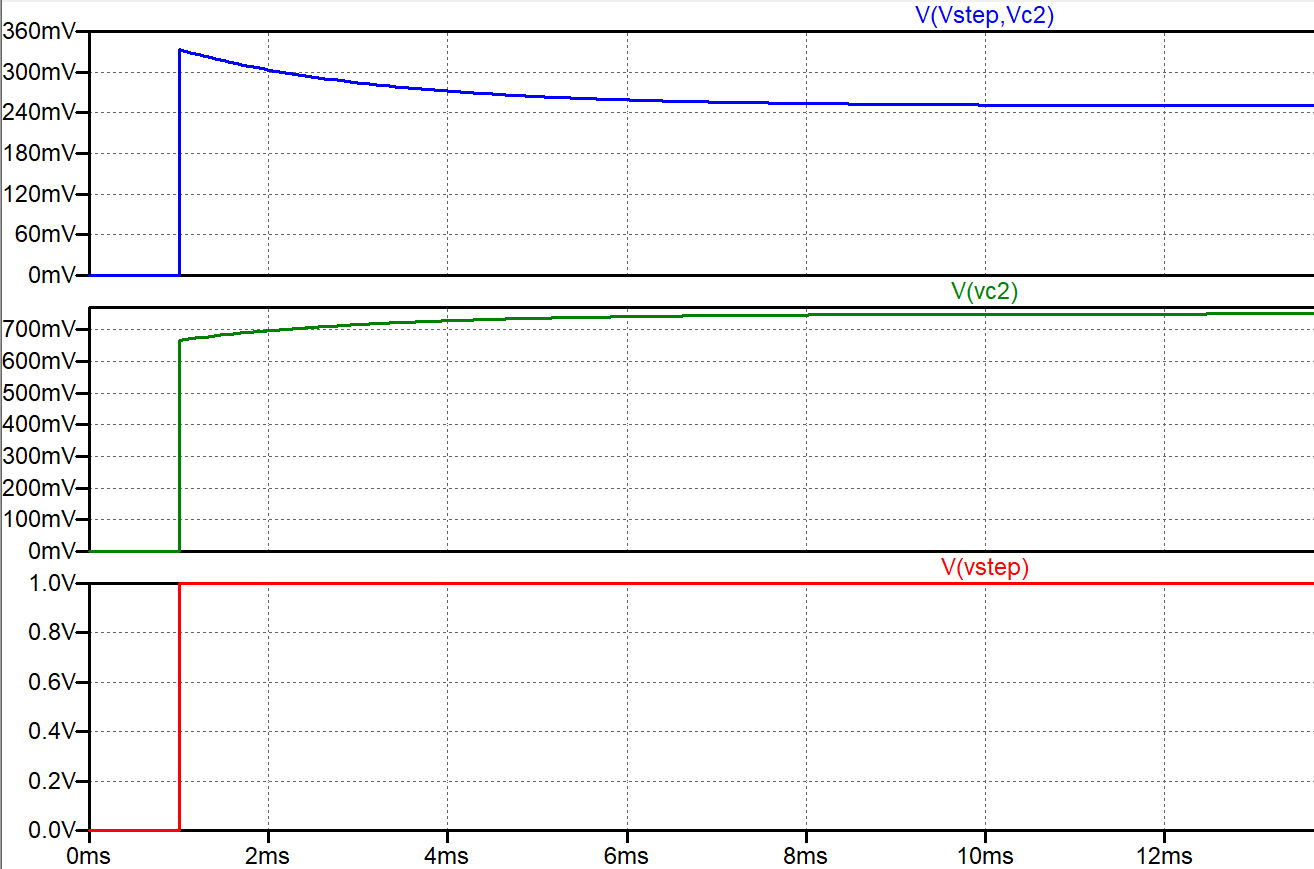Feel free to comment if you have any questions/suggestions.